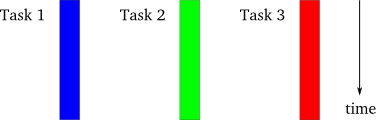## What is asynchronous Programming?asynchtomous execution

Two main difference between normal threaded system and asynchronous system are:

• For threaded execution each thread has its own controller, however for asynchronous system there is only one thread controller.
• Threaded execution does not give the control of ending, starting, changing to user. It is mainly controlled by the operating system internals. On the other side asynchronous execution need some explicit command to interleave one execution to other. It is more in control in the programmer’s perspective.

## Using Semaphore in C coding (Posix)

Semaphore is used for dealing with the synchronisation problem between processes and threads. It is well defined library structure that comes with the POSIX libraries.

Here a example that have a thread synchronisation problem between two threads. Here is the code.

``` #include <pthread.h> #include <stdio.h> #include <stdlib.h> #define UPTO 10000000 int count = 0; void * ThreadAdd(void * a) { int i, tmp; for(i = 0; i < UPTO; i++) { sem_wait(&mutex); tmp = count;      /* copy the global count locally */ tmp = tmp+1;      /* increment the local copy */ count = tmp;      /* store the local value into the global count */ //printf("The count is %d in %dn", count, (int)pthread_self()); sem_post(&mutex); } } int main(int argc, char * argv[]) { pthread_t tid1, tid2; if(pthread_create(&tid1, NULL, ThreadAdd, NULL)) { printf("n ERROR creating thread 1"); exit(1); } if(pthread_create(&tid2, NULL, ThreadAdd, NULL)) { printf("n ERROR creating thread 2"); exit(1); } if(pthread_join(tid1, NULL))    /* wait for the thread 1 to finish */ { printf("n ERROR joining thread"); exit(1); } if(pthread_join(tid2, NULL))        /* wait for the thread 2 to finish */ { printf("n ERROR joining thread"); exit(1); } if (count < 2 * UPTO) printf("n BOOM! count is [%d], should be %dn", count, 2*UPTO); else printf("n OK! count is [%d]n", count); pthread_exit(NULL); } ```

This code will have the synchronisation problem when you use it since the shared variable count will be updated with two threads unexpectedly. Consider the “for” section of the function “ThreadAdd”. In that section count variable is updated but this increment and update action is not atomic operation (that means, it needs multiple system calls in low level) so in the middle of the for loop the other thread can get the control and continue its execution. As a result it causes unexpected result. Let’s analysis execution flow of the threads.

initially “count = 1”

tmp = count;
tmp = tmp+1
count = tmp //(count = 2)

tmp = count;
tmp = tmp+1
count = tmp  //(count = 2)  Two for loops the give same result for count. So result is 2 instead of 3

For this kind of problems we can easily use Semaphore. Here the basic functions:

sem_t sem_name; //create variable

sem_init(&sem_name, Flag, Init_val) // initialise the semaphore var. “Flag” decides whether it is shared by processes. “Init_val” is the initial value of the semaphore.

sem_wait(&sem_name);//waits while semaphore value will  be positive, if value is positive it makes the semaphore negative again to make he other processes waiting.

sem_post(&sem_name);//increment the semaphore value, so one of the waiting thread can continue its operations.

sem_destroy(&sem_name);

Here the solution of the above code with semaphore:

```; #include <pthread.h> #include <unistd.h> #include <sys/types.h> #include <semaphore.h> #include <stdio.h> #include <stdlib.h> #define UPTO 10000000 int count = 0; sem_t mutex; void * ThreadAdd(void * a) { int i, tmp; for(i = 0; i < UPTO; i++) { sem_wait(&mutex); tmp = count;      /* copy the global count locally */ tmp = tmp+1;      /* increment the local copy */ count = tmp;      /* store the local value into the global count */ //printf("The count is %d in %dn", count, (int)pthread_self()); sem_post(&mutex); } } int main(int argc, char * argv[]) { pthread_t tid1, tid2; sem_init(&mutex, 0, 1); if(pthread_create(&tid1, NULL, ThreadAdd, NULL)) { printf("n ERROR creating thread 1"); exit(1); } if(pthread_create(&tid2, NULL, ThreadAdd, NULL)) { printf("n ERROR creating thread 2"); exit(1); } if(pthread_join(tid1, NULL))    /* wait for the thread 1 to finish */ { printf("n ERROR joining thread"); exit(1); } if(pthread_join(tid2, NULL))        /* wait for the thread 2 to finish */ { printf("n ERROR joining thread"); exit(1); } if (count < 2 * UPTO) printf("n BOOM! count is [%d], should be %dn", count, 2*UPTO); else printf("n OK! count is [%d]n", count); sem_destroy(&mutex); pthread_exit(NULL); } ```